# 2.5. Decomposing signals in components (matrix factorization problems)¶

## 2.5.1. Principal component analysis (PCA)¶

### 2.5.1.1. Exact PCA and probabilistic interpretation¶

PCA is used to decompose a multivariate dataset in a set of successive orthogonal components that explain a maximum amount of the variance. In scikit-learn, PCA is implemented as a transformer object that learns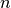components in its fit method, and can be used on new data to project it on these components.

The optional parameter whiten=True makes it possible to project the data onto the singular space while scaling each component to unit variance. This is often useful if the models down-stream make strong assumptions on the isotropy of the signal: this is for example the case for Support Vector Machines with the RBF kernel and the K-Means clustering algorithm.

Below is an example of the iris dataset, which is comprised of 4 features, projected on the 2 dimensions that explain most variance:

The PCA object also provides a probabilistic interpretation of the PCA that can give a likelihood of data based on the amount of variance it explains. As such it implements a score method that can be used in cross-validation:

### 2.5.1.2. Incremental PCA¶

The PCA object is very useful, but has certain limitations for large datasets. The biggest limitation is that PCA only supports batch processing, which means all of the data to be processed must fit in main memory. The IncrementalPCA object uses a different form of processing and allows for partial computations which almost exactly match the results of PCA while processing the data in a minibatch fashion. IncrementalPCA makes it possible to implement out-of-core Principal Component Analysis either by:

• Using its partial_fit method on chunks of data fetched sequentially from the local hard drive or a network database.
• Calling its fit method on a memory mapped file using numpy.memmap.

IncrementalPCA only stores estimates of component and noise variances, in order update explained_variance_ratio_ incrementally. This is why memory usage depends on the number of samples per batch, rather than the number of samples to be processed in the dataset.

Examples:

### 2.5.1.3. PCA using randomized SVD¶

It is often interesting to project data to a lower-dimensional space that preserves most of the variance, by dropping the singular vector of components associated with lower singular values.

For instance, if we work with 64x64 pixel gray-level pictures for face recognition, the dimensionality of the data is 4096 and it is slow to train an RBF support vector machine on such wide data. Furthermore we know that the intrinsic dimensionality of the data is much lower than 4096 since all pictures of human faces look somewhat alike. The samples lie on a manifold of much lower dimension (say around 200 for instance). The PCA algorithm can be used to linearly transform the data while both reducing the dimensionality and preserve most of the explained variance at the same time.

The class PCA used with the optional parameter svd_solver='randomized' is very useful in that case: since we are going to drop most of the singular vectors it is much more efficient to limit the computation to an approximated estimate of the singular vectors we will keep to actually perform the transform.

For instance, the following shows 16 sample portraits (centered around 0.0) from the Olivetti dataset. On the right hand side are the first 16 singular vectors reshaped as portraits. Since we only require the top 16 singular vectors of a dataset with size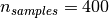and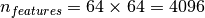, the computation time is less than 1s:

Note: with the optional parameter svd_solver='randomized', we also need to give PCA the size of the lower-dimensional space n_components as a mandatory input parameter.

If we note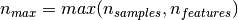and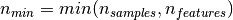, the time complexity of the randomized PCA is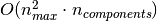instead of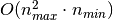for the exact method implemented in PCA.

The memory footprint of randomized PCA is also proportional to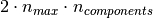instead of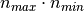for the exact method.

Note: the implementation of inverse_transform in PCA with svd_solver='randomized' is not the exact inverse transform of transform even when whiten=False (default).

References:

### 2.5.1.4. Kernel PCA¶

KernelPCA is an extension of PCA which achieves non-linear dimensionality reduction through the use of kernels (see Pairwise metrics, Affinities and Kernels). It has many applications including denoising, compression and structured prediction (kernel dependency estimation). KernelPCA supports both transform and inverse_transform.

Examples:

### 2.5.1.5. Sparse principal components analysis (SparsePCA and MiniBatchSparsePCA)¶

SparsePCA is a variant of PCA, with the goal of extracting the set of sparse components that best reconstruct the data.

Mini-batch sparse PCA (MiniBatchSparsePCA) is a variant of SparsePCA that is faster but less accurate. The increased speed is reached by iterating over small chunks of the set of features, for a given number of iterations.

Principal component analysis (PCA) has the disadvantage that the components extracted by this method have exclusively dense expressions, i.e. they have non-zero coefficients when expressed as linear combinations of the original variables. This can make interpretation difficult. In many cases, the real underlying components can be more naturally imagined as sparse vectors; for example in face recognition, components might naturally map to parts of faces.

Sparse principal components yields a more parsimonious, interpretable representation, clearly emphasizing which of the original features contribute to the differences between samples.

The following example illustrates 16 components extracted using sparse PCA from the Olivetti faces dataset. It can be seen how the regularization term induces many zeros. Furthermore, the natural structure of the data causes the non-zero coefficients to be vertically adjacent. The model does not enforce this mathematically: each component is a vector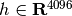, and there is no notion of vertical adjacency except during the human-friendly visualization as 64x64 pixel images. The fact that the components shown below appear local is the effect of the inherent structure of the data, which makes such local patterns minimize reconstruction error. There exist sparsity-inducing norms that take into account adjacency and different kinds of structure; see [Jen09] for a review of such methods. For more details on how to use Sparse PCA, see the Examples section, below.

Note that there are many different formulations for the Sparse PCA problem. The one implemented here is based on [Mrl09] . The optimization problem solved is a PCA problem (dictionary learning) with an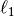penalty on the components: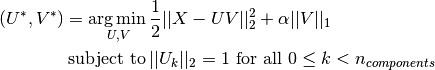The sparsity-inducingnorm also prevents learning components from noise when few training samples are available. The degree of penalization (and thus sparsity) can be adjusted through the hyperparameter alpha. Small values lead to a gently regularized factorization, while larger values shrink many coefficients to zero.

Note

While in the spirit of an online algorithm, the class MiniBatchSparsePCA does not implement partial_fit because the algorithm is online along the features direction, not the samples direction.

Examples:

References:

 [Mrl09] “Online Dictionary Learning for Sparse Coding” J. Mairal, F. Bach, J. Ponce, G. Sapiro, 2009
 [Jen09] “Structured Sparse Principal Component Analysis” R. Jenatton, G. Obozinski, F. Bach, 2009

## 2.5.2. Truncated singular value decomposition and latent semantic analysis¶

TruncatedSVD implements a variant of singular value decomposition (SVD) that only computes the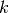largest singular values, whereis a user-specified parameter.

When truncated SVD is applied to term-document matrices (as returned by CountVectorizer or TfidfVectorizer), this transformation is known as latent semantic analysis (LSA), because it transforms such matrices to a “semantic” space of low dimensionality. In particular, LSA is known to combat the effects of synonymy and polysemy (both of which roughly mean there are multiple meanings per word), which cause term-document matrices to be overly sparse and exhibit poor similarity under measures such as cosine similarity.

Note

LSA is also known as latent semantic indexing, LSI, though strictly that refers to its use in persistent indexes for information retrieval purposes.

Mathematically, truncated SVD applied to training samples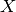produces a low-rank approximation: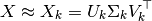After this operation,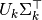is the transformed training set withfeatures (called n_components in the API).

To also transform a test set, we multiply it with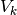: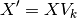Note

Most treatments of LSA in the natural language processing (NLP) and information retrieval (IR) literature swap the axes of the matrixso that it has shape n_features × n_samples. We present LSA in a different way that matches the scikit-learn API better, but the singular values found are the same.

TruncatedSVD is very similar to PCA, but differs in that it works on sample matricesdirectly instead of their covariance matrices. When the columnwise (per-feature) means ofare subtracted from the feature values, truncated SVD on the resulting matrix is equivalent to PCA. In practical terms, this means that the TruncatedSVD transformer accepts scipy.sparse matrices without the need to densify them, as densifying may fill up memory even for medium-sized document collections.

While the TruncatedSVD transformer works with any (sparse) feature matrix, using it on tf–idf matrices is recommended over raw frequency counts in an LSA/document processing setting. In particular, sublinear scaling and inverse document frequency should be turned on (sublinear_tf=True, use_idf=True) to bring the feature values closer to a Gaussian distribution, compensating for LSA’s erroneous assumptions about textual data.

References:

## 2.5.3. Dictionary Learning¶

### 2.5.3.1. Sparse coding with a precomputed dictionary¶

The SparseCoder object is an estimator that can be used to transform signals into sparse linear combination of atoms from a fixed, precomputed dictionary such as a discrete wavelet basis. This object therefore does not implement a fit method. The transformation amounts to a sparse coding problem: finding a representation of the data as a linear combination of as few dictionary atoms as possible. All variations of dictionary learning implement the following transform methods, controllable via the transform_method initialization parameter:

Thresholding is very fast but it does not yield accurate reconstructions. They have been shown useful in literature for classification tasks. For image reconstruction tasks, orthogonal matching pursuit yields the most accurate, unbiased reconstruction.

The dictionary learning objects offer, via the split_code parameter, the possibility to separate the positive and negative values in the results of sparse coding. This is useful when dictionary learning is used for extracting features that will be used for supervised learning, because it allows the learning algorithm to assign different weights to negative loadings of a particular atom, from to the corresponding positive loading.

The split code for a single sample has length 2 * n_components and is constructed using the following rule: First, the regular code of length n_components is computed. Then, the first n_components entries of the split_code are filled with the positive part of the regular code vector. The second half of the split code is filled with the negative part of the code vector, only with a positive sign. Therefore, the split_code is non-negative.

### 2.5.3.2. Generic dictionary learning¶

Dictionary learning (DictionaryLearning) is a matrix factorization problem that amounts to finding a (usually overcomplete) dictionary that will perform good at sparsely encoding the fitted data.

Representing data as sparse combinations of atoms from an overcomplete dictionary is suggested to be the way the mammal primary visual cortex works. Consequently, dictionary learning applied on image patches has been shown to give good results in image processing tasks such as image completion, inpainting and denoising, as well as for supervised recognition tasks.

Dictionary learning is an optimization problem solved by alternatively updating the sparse code, as a solution to multiple Lasso problems, considering the dictionary fixed, and then updating the dictionary to best fit the sparse code.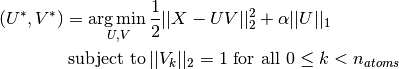After using such a procedure to fit the dictionary, the transform is simply a sparse coding step that shares the same implementation with all dictionary learning objects (see Sparse coding with a precomputed dictionary).

The following image shows how a dictionary learned from 4x4 pixel image patches extracted from part of the image of a raccoon face looks like.

References:

### 2.5.3.3. Mini-batch dictionary learning¶

MiniBatchDictionaryLearning implements a faster, but less accurate version of the dictionary learning algorithm that is better suited for large datasets.

By default, MiniBatchDictionaryLearning divides the data into mini-batches and optimizes in an online manner by cycling over the mini-batches for the specified number of iterations. However, at the moment it does not implement a stopping condition.

The estimator also implements partial_fit, which updates the dictionary by iterating only once over a mini-batch. This can be used for online learning when the data is not readily available from the start, or for when the data does not fit into the memory.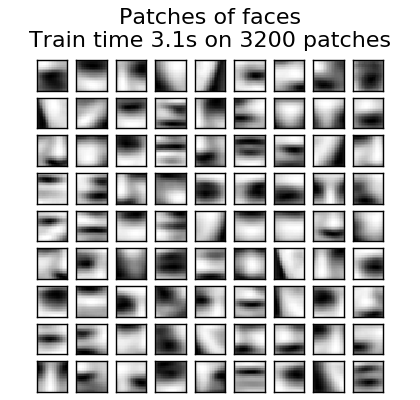Clustering for dictionary learning

Note that when using dictionary learning to extract a representation (e.g. for sparse coding) clustering can be a good proxy to learn the dictionary. For instance the MiniBatchKMeans estimator is computationally efficient and implements on-line learning with a partial_fit method.

## 2.5.4. Factor Analysis¶

In unsupervised learning we only have a dataset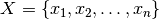. How can this dataset be described mathematically? A very simple continuous latent variable model foris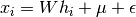The vector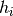is called “latent” because it is unobserved.is considered a noise term distributed according to a Gaussian with mean 0 and covariance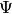(i.e.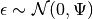),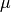is some arbitrary offset vector. Such a model is called “generative” as it describes how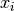is generated from. If we use all the‘s as columns to form a matrix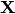and all the‘s as columns of a matrix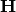then we can write (with suitably defined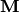and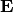):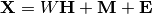In other words, we decomposed matrix.

Ifis given, the above equation automatically implies the following probabilistic interpretation: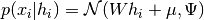For a complete probabilistic model we also need a prior distribution for the latent variable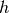. The most straightforward assumption (based on the nice properties of the Gaussian distribution) is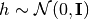. This yields a Gaussian as the marginal distribution of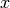: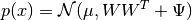Now, without any further assumptions the idea of having a latent variablewould be superfluous –can be completely modelled with a mean and a covariance. We need to impose some more specific structure on one of these two parameters. A simple additional assumption regards the structure of the error covariance:

•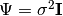: This assumption leads to the probabilistic model of PCA.
•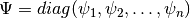: This model is called FactorAnalysis, a classical statistical model. The matrix W is sometimes called the “factor loading matrix”.

Both models essentially estimate a Gaussian with a low-rank covariance matrix. Because both models are probabilistic they can be integrated in more complex models, e.g. Mixture of Factor Analysers. One gets very different models (e.g. FastICA) if non-Gaussian priors on the latent variables are assumed.

Factor analysis can produce similar components (the columns of its loading matrix) to PCA. However, one can not make any general statements about these components (e.g. whether they are orthogonal):

The main advantage for Factor Analysis (over PCA is that it can model the variance in every direction of the input space independently (heteroscedastic noise):

This allows better model selection than probabilistic PCA in the presence of heteroscedastic noise:

## 2.5.5. Independent component analysis (ICA)¶

Independent component analysis separates a multivariate signal into additive subcomponents that are maximally independent. It is implemented in scikit-learn using the Fast ICA algorithm. Typically, ICA is not used for reducing dimensionality but for separating superimposed signals. Since the ICA model does not include a noise term, for the model to be correct, whitening must be applied. This can be done internally using the whiten argument or manually using one of the PCA variants.

It is classically used to separate mixed signals (a problem known as blind source separation), as in the example below:

ICA can also be used as yet another non linear decomposition that finds components with some sparsity:

## 2.5.6. Non-negative matrix factorization (NMF or NNMF)¶

NMF is an alternative approach to decomposition that assumes that the data and the components are non-negative. NMF can be plugged in instead of PCA or its variants, in the cases where the data matrix does not contain negative values. It finds a decomposition of samplesinto two matrices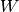and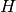of non-negative elements, by optimizing for the squared Frobenius norm: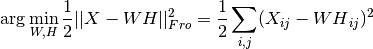This norm is an obvious extension of the Euclidean norm to matrices. (Other optimization objectives have been suggested in the NMF literature, in particular Kullback-Leibler divergence, but these are not currently implemented.)

Unlike PCA, the representation of a vector is obtained in an additive fashion, by superimposing the components, without subtracting. Such additive models are efficient for representing images and text.

It has been observed in [Hoyer, 04] that, when carefully constrained, NMF can produce a parts-based representation of the dataset, resulting in interpretable models. The following example displays 16 sparse components found by NMF from the images in the Olivetti faces dataset, in comparison with the PCA eigenfaces.

The init attribute determines the initialization method applied, which has a great impact on the performance of the method. NMF implements the method Nonnegative Double Singular Value Decomposition. NNDSVD is based on two SVD processes, one approximating the data matrix, the other approximating positive sections of the resulting partial SVD factors utilizing an algebraic property of unit rank matrices. The basic NNDSVD algorithm is better fit for sparse factorization. Its variants NNDSVDa (in which all zeros are set equal to the mean of all elements of the data), and NNDSVDar (in which the zeros are set to random perturbations less than the mean of the data divided by 100) are recommended in the dense case.

NMF can also be initialized with correctly scaled random non-negative matrices by setting init="random". An integer seed or a RandomState can also be passed to random_state to control reproducibility.

In NMF, L1 and L2 priors can be added to the loss function in order to regularize the model. The L2 prior uses the Frobenius norm, while the L1 prior uses an elementwise L1 norm. As in ElasticNet, we control the combination of L1 and L2 with the l1_ratio (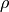) parameter, and the intensity of the regularization with the alpha (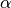) parameter. Then the priors terms are: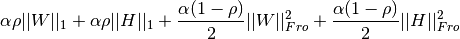and the regularized objective function is: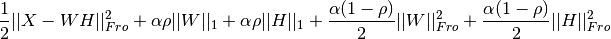NMF regularizes both W and H. The public function non_negative_factorization allows a finer control through the regularization attribute, and may regularize only W, only H, or both.

## 2.5.7. Latent Dirichlet Allocation (LDA)¶

Latent Dirichlet Allocation is a generative probabilistic model for collections of discrete dataset such as text corpora. It is also a topic model that is used for discovering abstract topics from a collection of documents.

The graphical model of LDA is a three-level Bayesian model: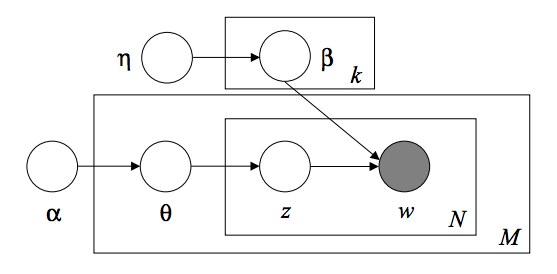When modeling text corpora, the model assumes the following generative process for a corpus with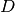documents and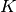topics:

1. For each topic, draw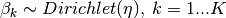2. For each document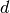, draw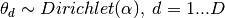3. For each wordin document:
1. Draw a topic index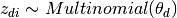2. Draw the observed word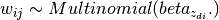For parameter estimation, the posterior distribution is: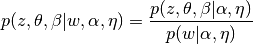Since the posterior is intractable, variational Bayesian method uses a simpler distribution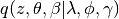to approximate it, and those variational parameters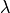,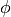,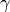are optimized to maximize the Evidence Lower Bound (ELBO):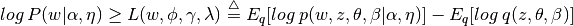Maximizing ELBO is equivalent to minimizing the Kullback-Leibler(KL) divergence between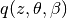and the true posterior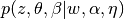.

LatentDirichletAllocation implements online variational Bayes algorithm and supports both online and batch update method. While batch method updates variational variables after each full pass through the data, online method updates variational variables from mini-batch data points.

Note

Although online method is guaranteed to converge to a local optimum point, the quality of the optimum point and the speed of convergence may depend on mini-batch size and attributes related to learning rate setting.

When LatentDirichletAllocation is applied on a “document-term” matrix, the matrix will be decomposed into a “topic-term” matrix and a “document-topic” matrix. While “topic-term” matrix is stored as components_ in the model, “document-topic” matrix can be calculated from transform method.

LatentDirichletAllocation also implements partial_fit method. This is used when data can be fetched sequentially.

References: